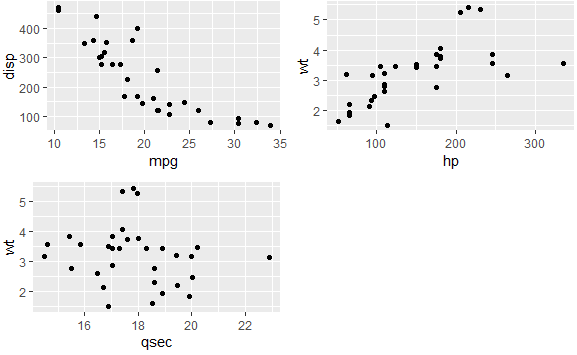3 views

This is cross-posted on the ggplot2 google group

My situation is that I'm working on a function that outputs an arbitrary number of plots (depending upon the input data supplied by the user). The function returns a list of n plots, and I'd like to lay those plots out in 2 x 2 formation. I'm struggling with the simultaneous problems of:

• How can I allow the flexibility to be handed an arbitrary (n) number of plots?
• How can I also specify I want them laid out 2 x 2

My current strategy uses grid.arrange from the gridExtra package. It's probably not optimal, especially since, and this is key, it totally doesn't work. Here's my commented sample code, experimenting with three plots:

library(ggplot2)

library(gridExtra)

x <- qplot(mpg, disp, data = mtcars)

y <- qplot(hp, wt, data = mtcars)

z <- qplot(qsec, wt, data = mtcars)

# A normal, plain-jane call to grid.arrange is fine for displaying all my plots

grid.arrange(x, y, z)

# But, for my purposes, I need a 2 x 2 layout. So the command below works acceptably.

grid.arrange(x, y, z, nrow = 2, ncol = 2)

# The problem is that the function I'm developing outputs a LIST of an arbitrary

# number plots, and I'd like to be able to plot every plot in the list on a 2 x 2

# laid-out page. I can at least plot a list of plots by constructing a do.call()

# expression, below. (Note: it totally even surprises me that this do.call expression

# DOES work. I'm astounded.)

plot.list <- list(x, y, z)

do.call(grid.arrange, plot.list)

# But now I need 2 x 2 pages. No problem, right? Since do.call() is taking a list of

# arguments, I'll just add my grid.layout arguments to the list. Since grid.arrange is

# supposed to pass layout arguments along to grid.layout anyway, this should work.

args.list <- c(plot.list, "nrow = 2", "ncol = 2")

# Except that the line below is going to fail, producing an "input must be grobs!"

# error

do.call(grid.arrange, args.list)

As I am wont to do, I humbly huddle in the corner, eagerly awaiting the sagacious feedback of a community far wiser than I. Especially if I'm making this harder than it needs to be.

by

To arrange the plots in 2 x 2 layout, you need to pass a named list to the do.call function as follows:

library(ggplot2)

library(gridExtra)

x <- qplot(mpg, disp, data = mtcars)

y <- qplot(hp, wt, data = mtcars)

z <- qplot(qsec, wt, data = mtcars)

args.list <- c(plot.list,list(nrow=2,ncol=2))

do.call(grid.arrange, args.list)

Output: